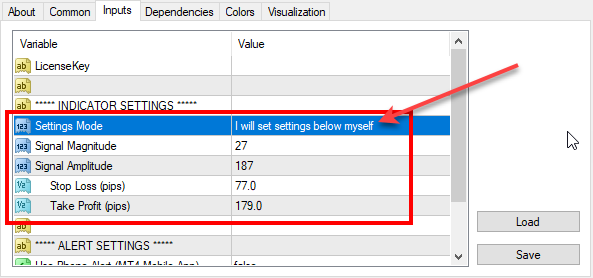# Re-optimized FxMagnetic indicator on EURUSD (2019-10-04)

We’ve reoptimized FxMagnetic indicator settings on EURUSD. Here are the latest parameters for all EURUSD timeframes that you should use for best MT4 indicator performance.To set parameters manually you need to switch the Settings Mode to: I will set settings below myself

Don’t have FxMagnetic indicator yet? You can buy FxMagnetic here.

## 1-minute chart:

Signal Magnitude = 10

Signal Amplitude = -180

Stop Loss (pips) = 20

Take Profit (pips) = 70

## 5-minute chart:

Signal Magnitude = 50

Signal Amplitude = -101

Stop Loss (pips) = 93

Take Profit (pips) = 133

## 15-minute chart:

Signal Magnitude = 92;

Signal Amplitude = -255;

Stop Loss (pips) = 104;

Take Profit (pips) = 105;

## 30-minute chart:

Signal Magnitude = 20;

Signal Amplitude = -137;

Stop Loss (pips) = 62;

Take Profit (pips) = 146;

## 1-hour chart:

Signal Magnitude = 20;

Signal Amplitude = -211;

Stop Loss (pips) = 94;

Take Profit (pips) = 91;

## 4-hour chart:

Signal Magnitude = 10;

Signal Amplitude = -110;

Stop Loss (pips) = 129;

Take Profit (pips) = 206;

## Daily chart:

Signal Magnitude = 68;

Signal Amplitude = -28;

Stop Loss (pips) = 330;

Take Profit (pips) = 512;

## Weekly chart:

Signal Magnitude = 10;

Ind0Param1 = -50;

Stop Loss (pips) = 320;

Take Profit (pips) = 1010;

## Monthly chart:

Signal Magnitude = 20;

Signal Amplitude = -80;

Stop Loss (pips) = 360;

Take Profit (pips) = 1000;

## FxMagnetic EURUSD v2.7 stats on 4th October, 2019

Below are the screenshots of FxMagnetic EURUSD stats from the previous 100 signals. This was taken on 2019-10-04 on the FxOpen Live ECN account. We re-optimized FxMagnetic EURUSD v2.7 on this broker so you’ll see the best signals with them.

Remember, past performance does not guarantee future results.

We do not know the future and none of the world’s trading algorithms know the future. All the stats above and trading signals are based on pure mathematical formulas and probability theory.

1.Joao Cunha on October 4, 2019 at 2:32 pm

Dear Sir,

when I changed the settings with the new parameters for M1, the winning rate and total profit didn`t change. Why is that? When we change parameters, the historical result should change?

•FxMagnetic on October 4, 2019 at 3:54 pm

Make sure in the indicator INPUTS tab you set this correctly:
Settings Mode = I will set settings below myself

2.Joao Cunha on October 4, 2019 at 2:36 pm

do you provide an EA for this indicator? if not, can you do it? the reason behind this is that being a part time trader it doesn`t make sense to trade this as I may loose the best signals.

•FxMagnetic on October 4, 2019 at 3:54 pm

Technically it is possible to turn FxMagnetic indicator into EA, but I will not do that because I created this product for manual Forex traders. Manual Forex traders observe each entry signal of FxMagnetic but if it does not align with their technical or fundamental analysis they might skip particular trading signals. The EA, in this case, would be worthless because EA cannot read the mind of every trader. If you are into automated trading then I invite you to our webinar at http://www.forexrobotacademy.com where you can learn how to create EAs yourself without programming.